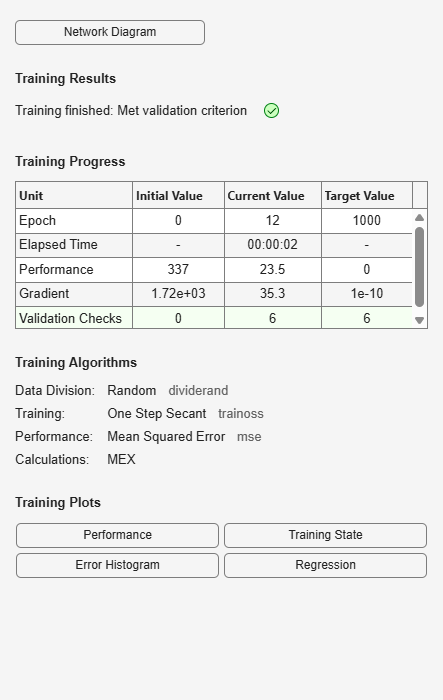# trainoss

One-step secant backpropagation

## Syntax

```net.trainFcn = 'trainoss' [net,tr] = train(net,...) ```

## Description

`trainoss` is a network training function that updates weight and bias values according to the one-step secant method.

`net.trainFcn = 'trainoss'` sets the network `trainFcn` property.

`[net,tr] = train(net,...)` trains the network with `trainoss`.

Training occurs according to `trainoss` training parameters, shown here with their default values:

 `net.trainParam.epochs` `1000` Maximum number of epochs to train `net.trainParam.goal` `0` Performance goal `net.trainParam.max_fail` `6` Maximum validation failures `net.trainParam.min_grad` `1e-10` Minimum performance gradient `net.trainParam.searchFcn` `'srchbac'` Name of line search routine to use `net.trainParam.show` `25` Epochs between displays (`NaN` for no displays) `net.trainParam.showCommandLine` `false` Generate command-line output `net.trainParam.showWindow` `true` Show training GUI `net.trainParam.time` `inf` Maximum time to train in seconds

Parameters related to line search methods (not all used for all methods):

 `net.trainParam.scal_tol` `20` Divide into `delta` to determine tolerance for linear search. `net.trainParam.alpha` `0.001` Scale factor that determines sufficient reduction in `perf` `net.trainParam.beta` `0.1` Scale factor that determines sufficiently large step size `net.trainParam.delta` `0.01` Initial step size in interval location step `net.trainParam.gama` `0.1` Parameter to avoid small reductions in performance, usually set to `0.1` (see `srch_cha`) `net.trainParam.low_lim` `0.1` Lower limit on change in step size `net.trainParam.up_lim` ` 0.5` Upper limit on change in step size `net.trainParam.maxstep` `100` Maximum step length `net.trainParam.minstep` `1.0e-6` Minimum step length `net.trainParam.bmax` `26` Maximum step size

## Network Use

You can create a standard network that uses `trainoss` with `feedforwardnet` or `cascadeforwardnet`. To prepare a custom network to be trained with `trainoss`:

1. Set `net.trainFcn` to `'trainoss'`. This sets `net.trainParam` to `trainoss`’s default parameters.

2. Set `net.trainParam` properties to desired values.

In either case, calling `train` with the resulting network trains the network with `trainoss`.

## Examples

collapse all

This example shows how to train a neural network using the `trainoss` train function.

Here a neural network is trained to predict body fat percentages.

```[x, t] = bodyfat_dataset; net = feedforwardnet(10, 'trainoss'); net = train(net, x, t);````y = net(x);`

collapse all

### One Step Secant Method

Because the BFGS algorithm requires more storage and computation in each iteration than the conjugate gradient algorithms, there is need for a secant approximation with smaller storage and computation requirements. The one step secant (OSS) method is an attempt to bridge the gap between the conjugate gradient algorithms and the quasi-Newton (secant) algorithms. This algorithm does not store the complete Hessian matrix; it assumes that at each iteration, the previous Hessian was the identity matrix. This has the additional advantage that the new search direction can be calculated without computing a matrix inverse.

The one step secant method is described in [Batt92]. This algorithm requires less storage and computation per epoch than the BFGS algorithm. It requires slightly more storage and computation per epoch than the conjugate gradient algorithms. It can be considered a compromise between full quasi-Newton algorithms and conjugate gradient algorithms.

## Algorithms

`trainoss` can train any network as long as its weight, net input, and transfer functions have derivative functions.

Backpropagation is used to calculate derivatives of performance `perf` with respect to the weight and bias variables `X`. Each variable is adjusted according to the following:

```X = X + a*dX; ```

where `dX` is the search direction. The parameter `a` is selected to minimize the performance along the search direction. The line search function `searchFcn` is used to locate the minimum point. The first search direction is the negative of the gradient of performance. In succeeding iterations the search direction is computed from the new gradient and the previous steps and gradients, according to the following formula:

```dX = -gX + Ac*X_step + Bc*dgX; ```

where `gX` is the gradient, `X_step` is the change in the weights on the previous iteration, and `dgX` is the change in the gradient from the last iteration. See Battiti (Neural Computation, Vol. 4, 1992, pp. 141–166) for a more detailed discussion of the one-step secant algorithm.

Training stops when any of these conditions occurs:

• The maximum number of `epochs` (repetitions) is reached.

• The maximum amount of `time` is exceeded.

• Performance is minimized to the `goal`.

• The performance gradient falls below `min_grad`.

• Validation performance (validation error) has increased more than `max_fail` times since the last time it decreased (when using validation).

## References

Battiti, R., “First and second order methods for learning: Between steepest descent and Newton’s method,” Neural Computation, Vol. 4, No. 2, 1992, pp. 141–166

## Version History

Introduced before R2006a